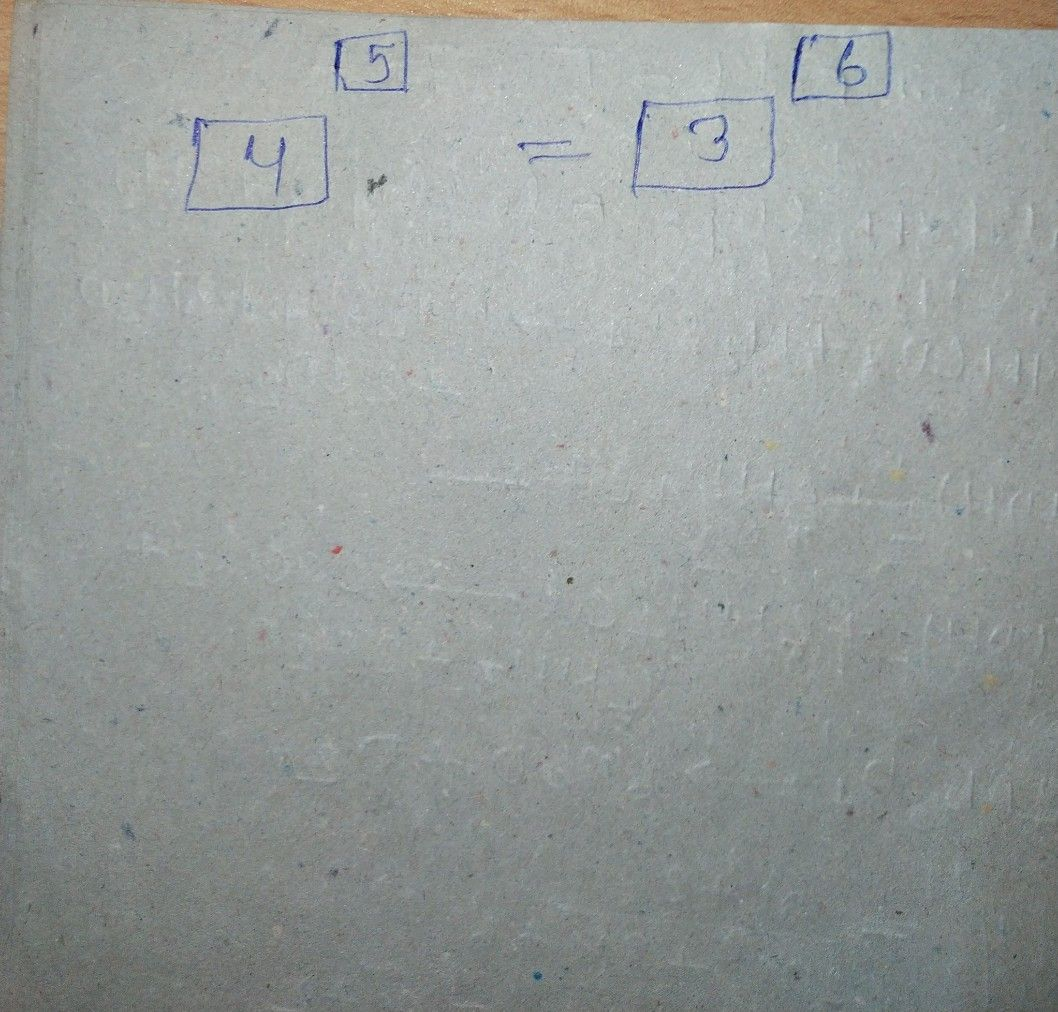Symbol
Problem$Directions$ Using the digits $0$ to $9,$ only once each, fill in the blanks to create an equation that is true. Show your work on the next board (use right arrow on $→$ top of screen to $sMitch\right)$ $\bar{1}$ $2$ $9$ $0$ $7$ $2$ $3$ $∠$ $x$ $5$ $\vec{6}$ $7$ $8$
7th-9th grade
Algebra
Search count: 135
SolutionQanda teacher - ShubhamSon